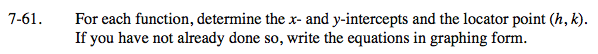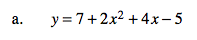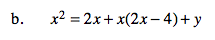Home > INT3 > Chapter 7 > Lesson 7.2.1 > Problem7-61

7-61.
1. For each function, determine the x‑ and y‑intercepts and the locator point (h, k). If you have not already done so, write the equations in graphing form. Homework Help ✎

1. y = 7 + 2x2 + 4x – 5

2. x2 = 2x + x(2x – 4) + yy-intercept: y = 7 + 2(0)2 + 4(0) − 5

x-intercept: 0 = 7 + 2x2 + 4x − 5

Since there is only one x-intercept, the vertex must be at the x-intercept.

y-intercept: (0, 2)
x-intercept: (−1, 0)
vertex: (−1, 0)
y = 2(x + 1)2Start by determining the x- and y-intercepts.

To find the x-coordinate of the vertex, average the x-intercepts.

To find the y-coordinate of the vertex, substitute the x-coordinate of the vertex into the given equation and solve for y.

Now write the equation in graphing form.